Econ. 153a Fall 1996 C. Sims

# Overlapping Generations Exercise

1. Calibrate our overlapping generations model as follows. Choose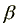to make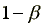match the ratio of labor income to gross output (GDP) in the US, taking some kind of average over 1950-96 (or a shorter period if that's necessary because of data limitations). You should be able to find this from the national income and product accounts. Choose as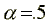. Choose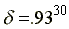(7% per year, converted to a 30-year rate). Choose 1+n to match the population growth rate over 1900-90, converted to a 30-year rate.
2. Find the implied steady state interest rate. (Note that the steady-state interest rate does not depend on A.) Is it a reasonable value? Check it against the average postwar US real interest rate, measured as the average nominal interest rate minus the average inflation rate. (This would be something like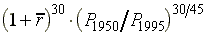, where the P's are values of the GDP deflator and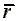is an average of nominal interest rates quoted at the usual annual rate.)
3. Suppose this economy was at its steady state in 1920, but that the changes in population size given by the numbers born in 1920, 1950, and 1980 constituted a pattern of deviations from the trend path of population for three "generations". Calculate how the relative per capita wages and utilities of the generations born at these three dates would vary. Assume the number in the 2010 generation return to the trend growth rate.

To do this exercise, you need to find data. Any source can be used. The Department of Commerce publishes the National Income and Product Accounts in the Survey of Current Business, which you should be able to find in the library. Probably all the data you need are available on the net at FRED, the Federal Reserve Bank of St. Louis data page and at the Bureau of the Census web site (http://www.census.gov/) for population.